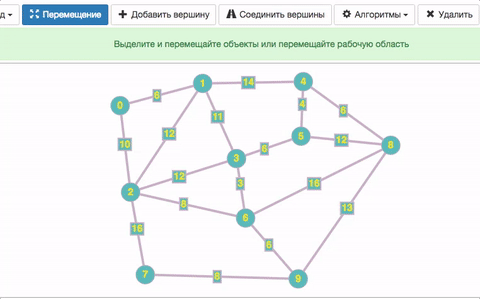Help

# Calculate vertexes degree

### Vertexes degree

Vertexes degree for undirected graph is the number of arches entering or coming from the vertex.

For directed graph Graph Online will calculate the number of arches coming from the vertex.

In order to use the algorithm choose “Algorithms” in the menu bar and then “Calculate vertexes degree”Vertexes degree will appear above every vertex. The vertexes of the same degree will be of the same colour.